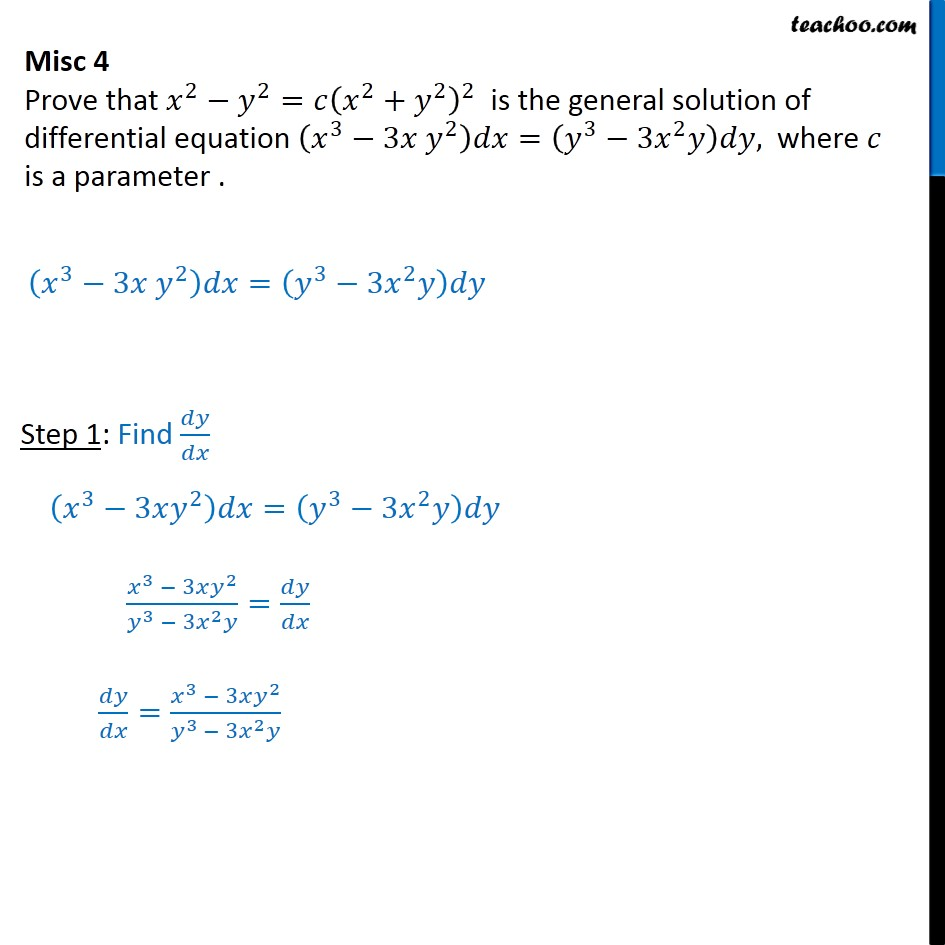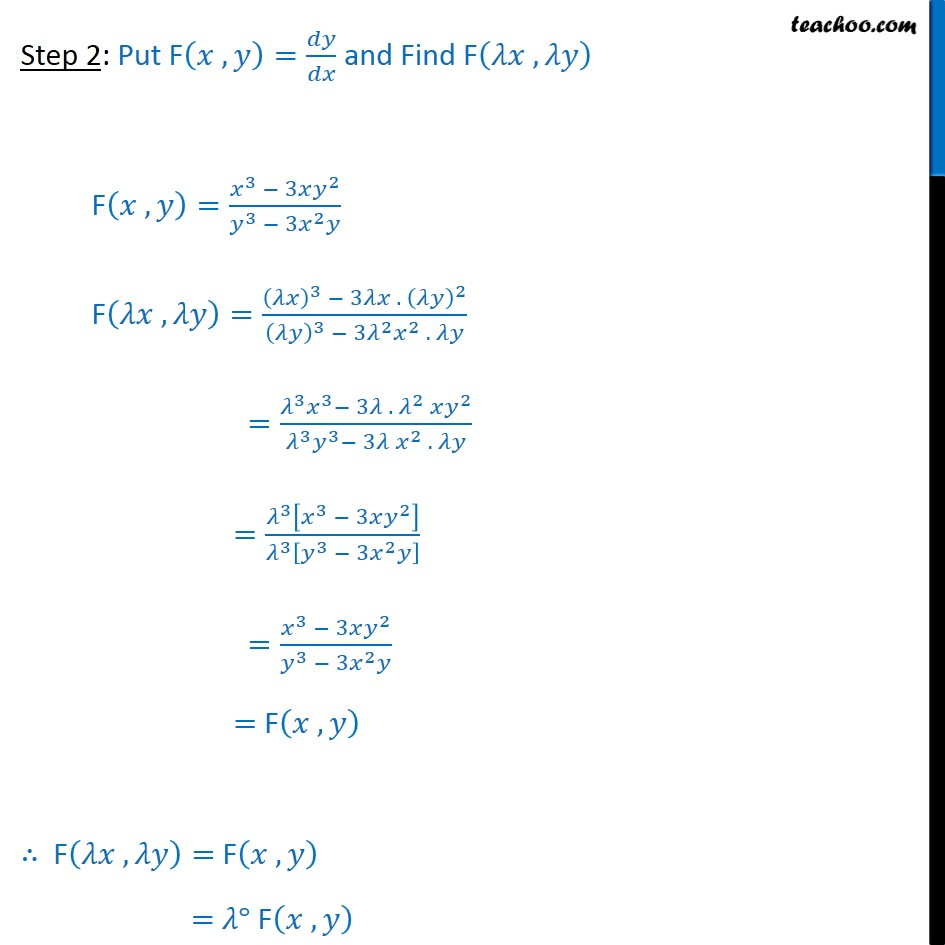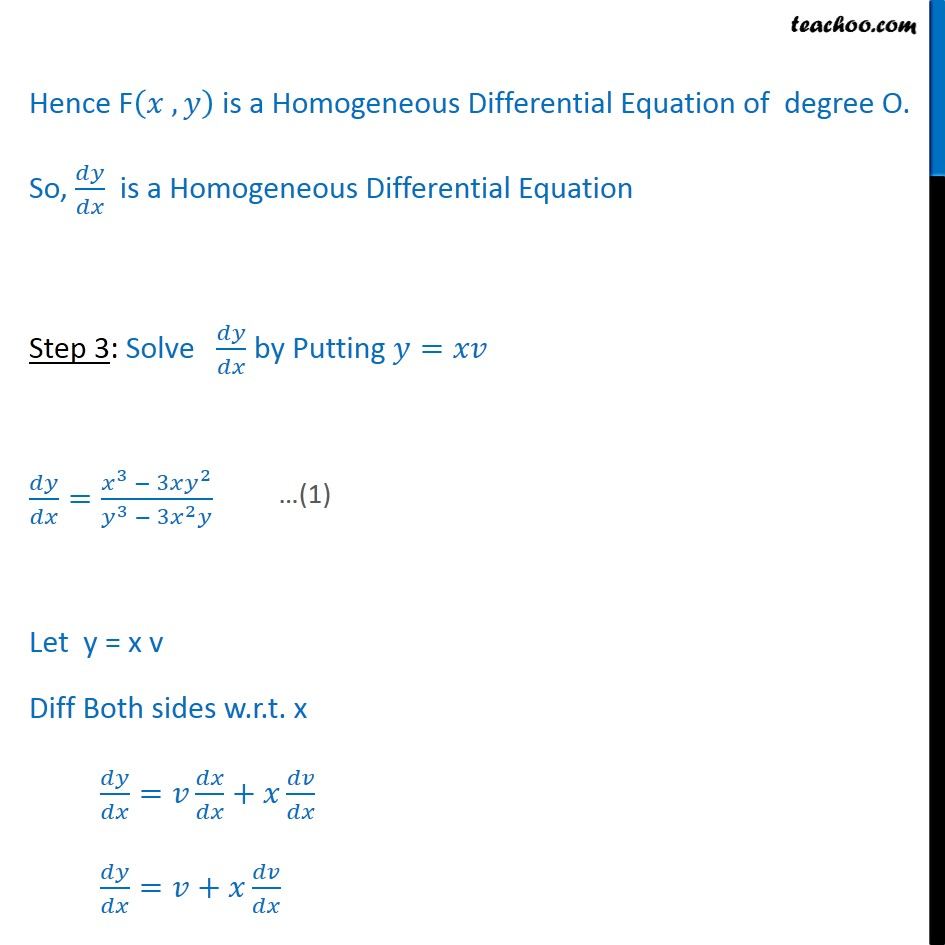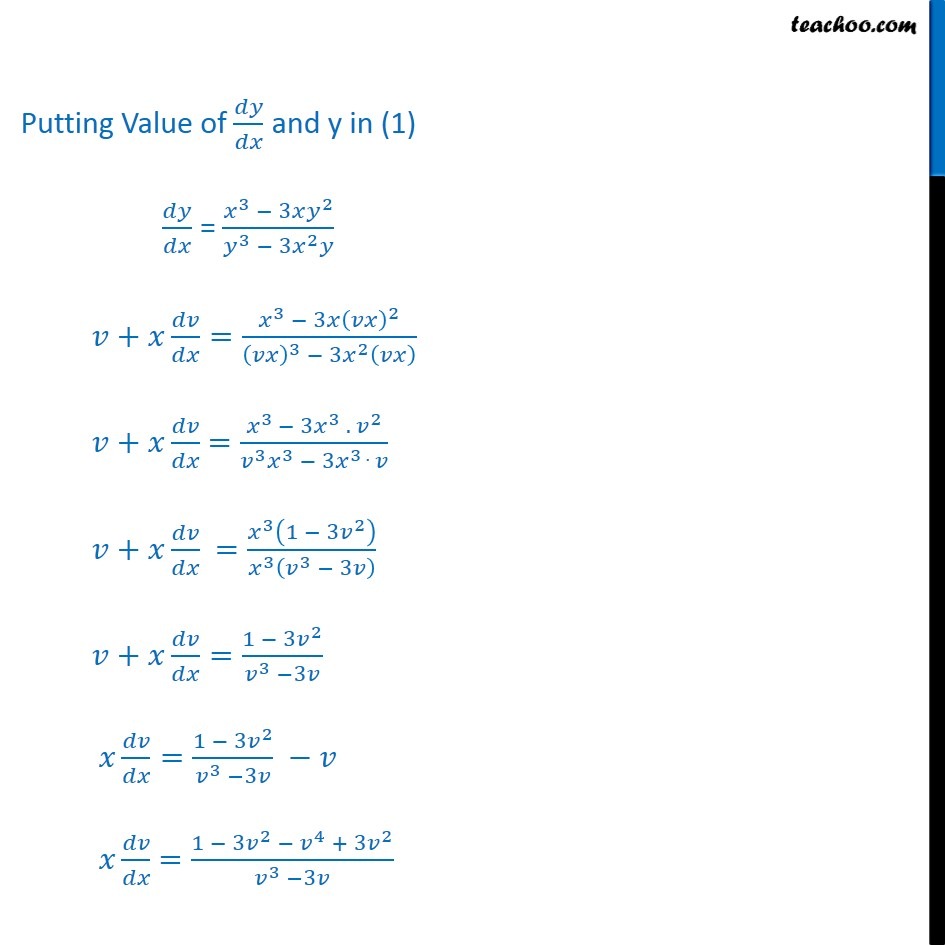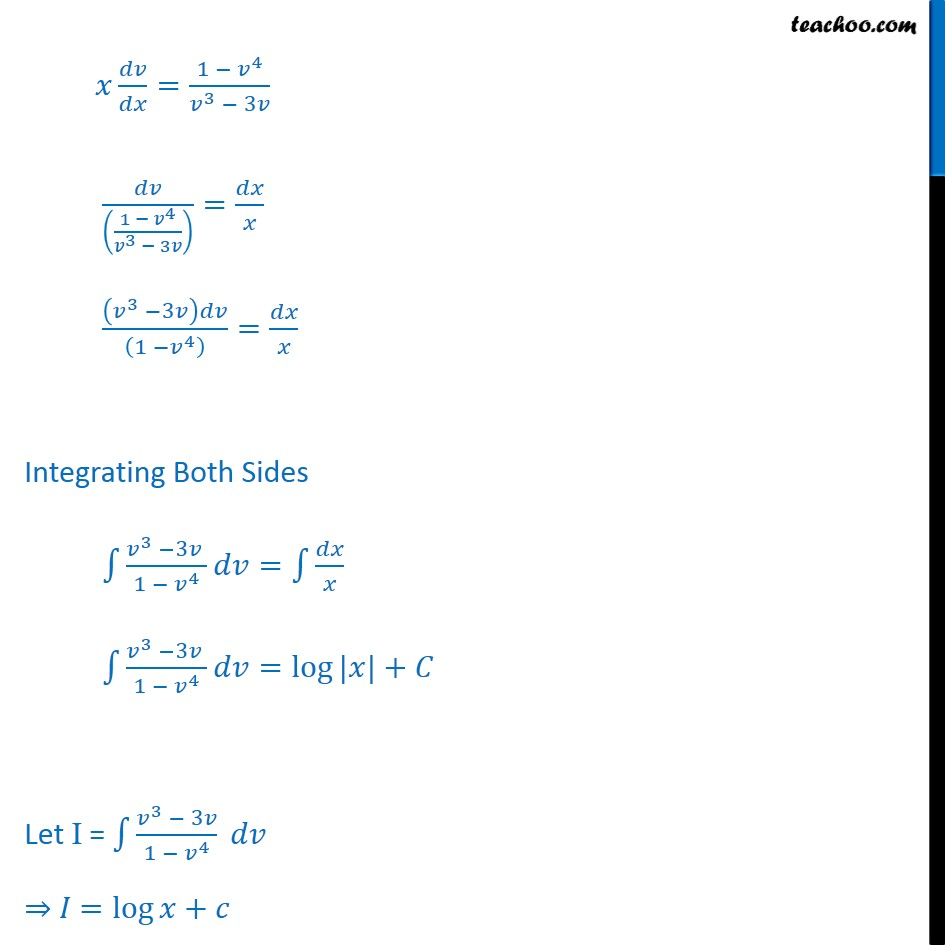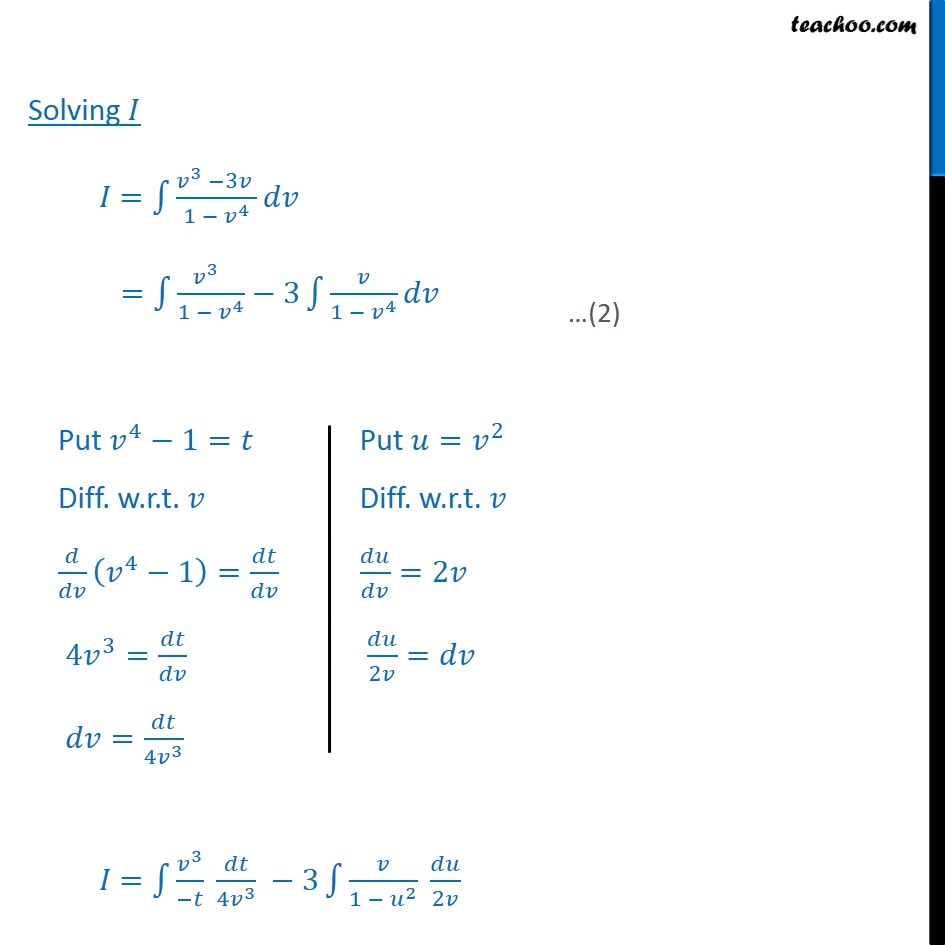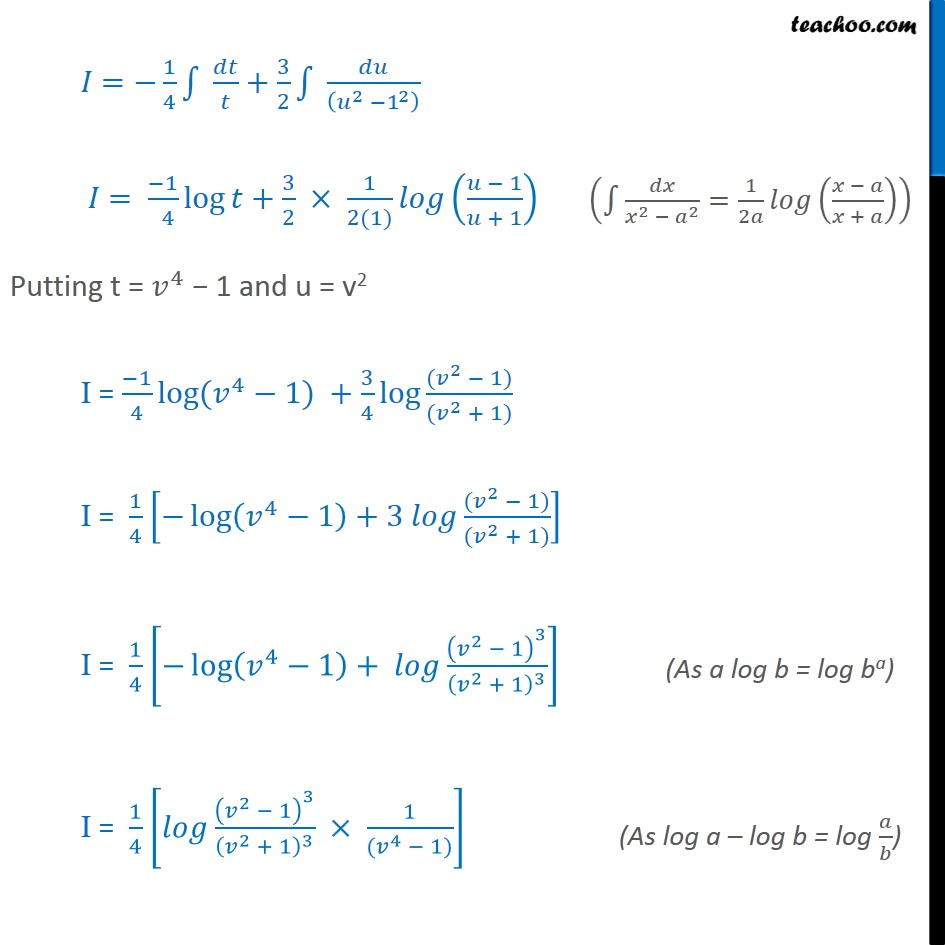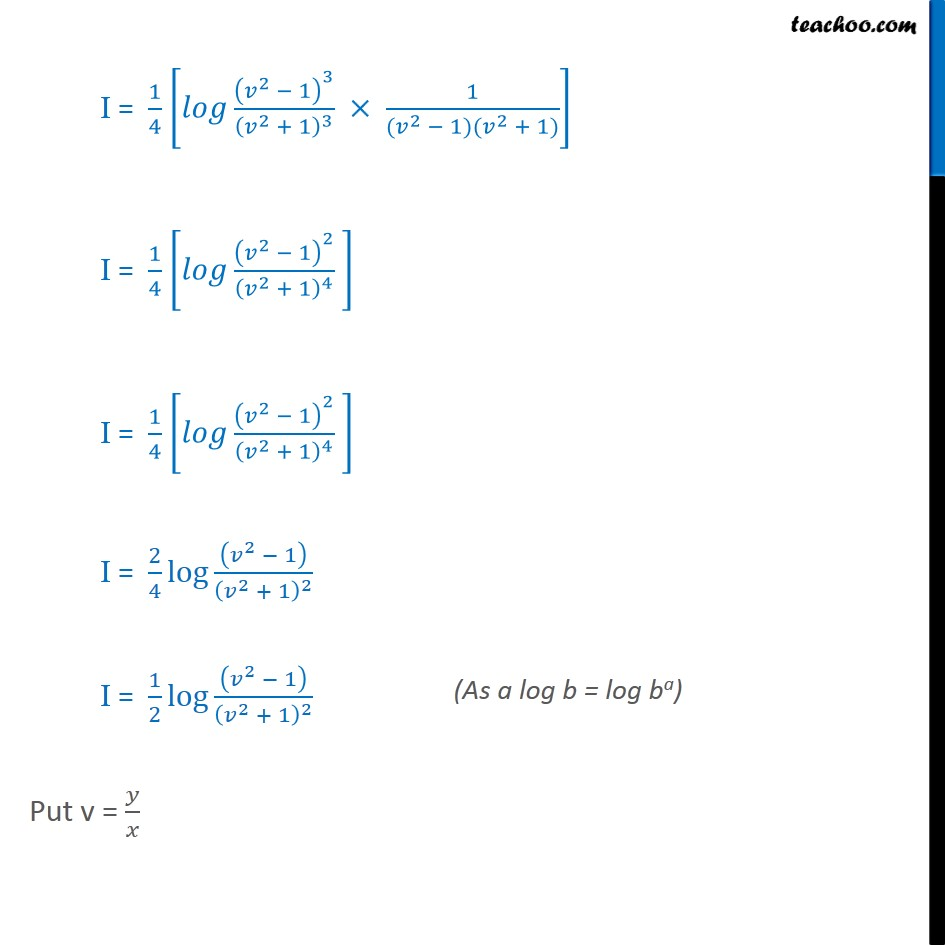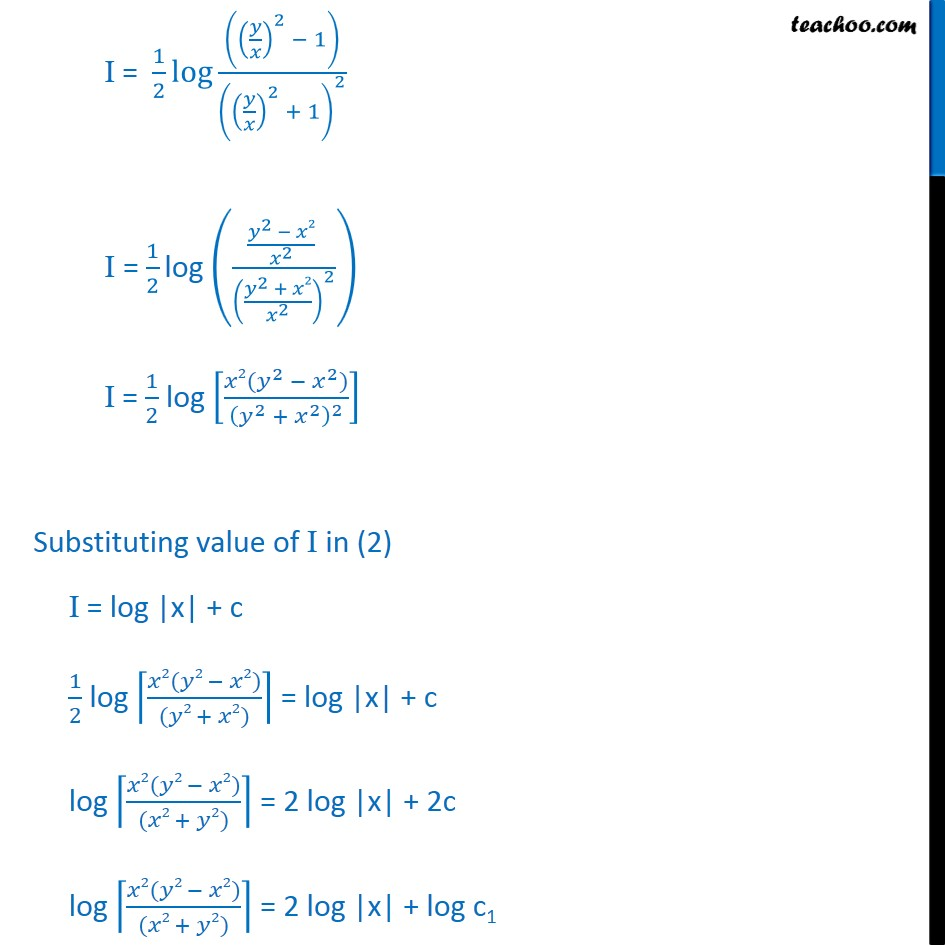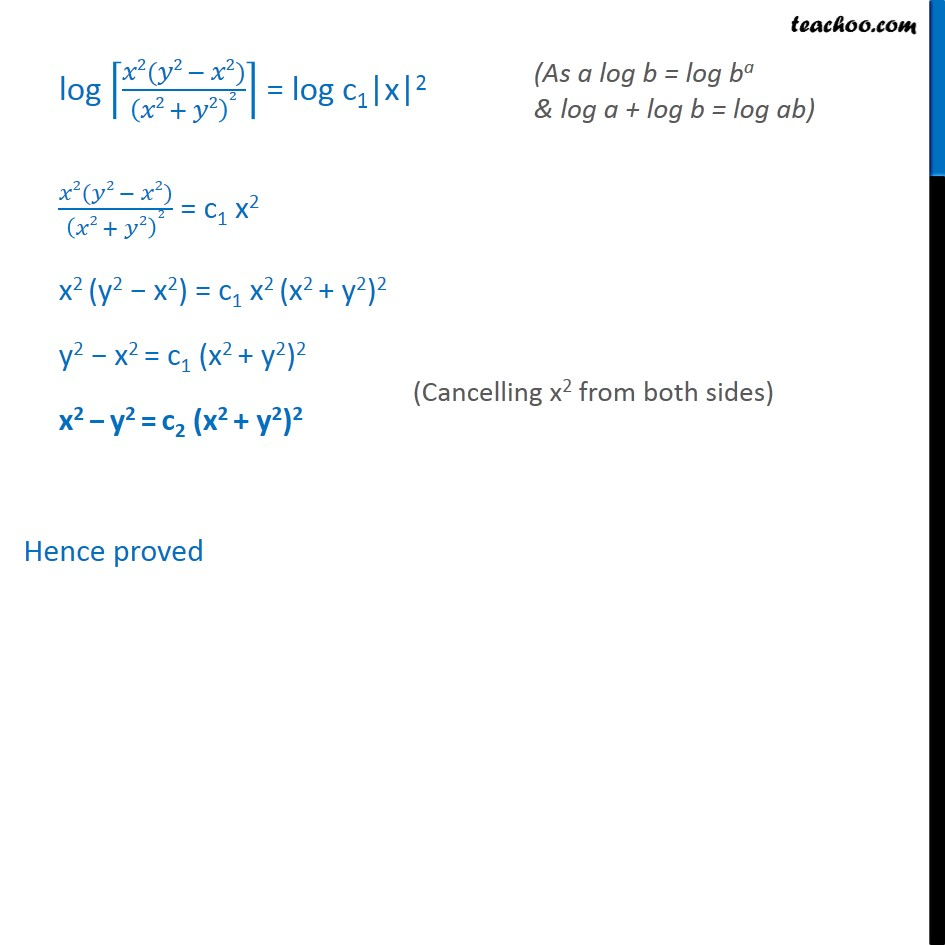1. Chapter 9 Class 12 Differential Equations
2. Serial order wise
3. Miscellaneous

Transcript

Misc 4 Prove that 2 2 = 2 + 2 2 is the general solution of differential equation 3 3 2 = 3 3 2 , where is a parameter . 3 3 2 = 3 3 2 Step 1: Find 3 3 2 = 3 3 2 3 3 2 3 3 2 = = 3 3 2 3 3 2 Step 2: Put F , = and Find F , F , = 3 3 2 3 3 2 F , = 3 3 . 2 3 3 2 2 . = 3 3 3 . 2 2 3 3 3 2 . = 3 3 3 2 3 3 3 2 = 3 3 2 3 3 2 = F , F , = F , = F , Hence F , is a Homogeneous Differential Equation of degree O. So, is a Homogeneous Differential Equation Step 3: Solve by Putting = = 3 3 2 3 3 2 Let y = x v Diff Both sides w.r.t. x = + = + Putting Value of and y in (1) = 3 3 2 3 3 2 + = 3 3 2 3 3 2 + = 3 3 3 . 2 3 3 3 3 . + = 3 1 3 2 3 3 3 + = 1 3 2 3 3 = 1 3 2 3 3 = 1 3 2 4 + 3 2 3 3 = 1 4 3 3 1 4 3 3 = 3 3 1 4 = Integrating Both Sides 3 3 1 4 = 3 3 1 4 = log | | + Let I = 3 3 1 4 = log + Solving = 3 3 1 4 = 3 1 4 3 1 4 = 3 4 3 3 1 2 2 = 1 4 + 3 2 2 1 2 = 1 4 log + 3 2 1 2(1) 1 + 1 Putting t = 4 1 and u = v2 I = 1 4 log ( 4 1) + 3 4 log ( 2 1) ( 2 + 1) I = 1 4 log 4 1 +3 ( 2 1) ( 2 + 1) I = 1 4 log 4 1 + 2 1 3 2 + 1 3 I = 1 4 2 1 3 2 + 1 3 1 ( 4 1) I = 1 4 2 1 3 2 + 1 3 1 ( 2 1)( 2 + 1) I = 1 4 2 1 2 2 + 1 4 I = 1 4 2 1 2 2 + 1 4 I = 2 4 log 2 1 2 + 1 2 I = 1 2 log 2 1 2 + 1 2 Put v = I = 1 2 log 2 1 2 + 1 2 I = 1 2 log 2 2 2 2 + 2 2 2 I = 1 2 log 2( 2 2 ) 2 + 2 2 Substituting value of I in (2) I = log |x| + c 1 2 log 2( 2 2) ( 2 + 2) = log |x| + c log 2( 2 2) ( 2 + 2) = 2 log |x| + 2c log 2( 2 2) ( 2 + 2) = 2 log |x| + log c1 log 2( 2 2) 2 + 2 2 = log c1|x|2 2( 2 2) 2 + 2 2 = c1 x2 x2 (y2 x2) = c1 x2 (x2 + y2)2 y2 x2 = c1 (x2 + y2)2 x2 y2 = c2 (x2 + y2)2 Hence proved

Miscellaneous